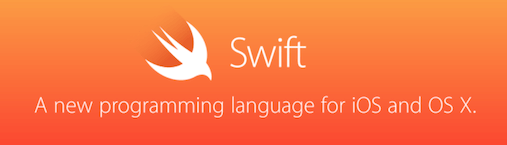Objective-C 确实很老了，感觉它就和 C++ 一样，历史包袱太重：本来就要兼容 C，还要不断添加新特性，支持各种编程范式，积重难返。

### TypeAlias 类型别名：类似 C++ 的 typedef 和 Golang 的 type

Swift 版本:

``typealias status = Int8``

C++ 版本：

``typedef status int``

Golang 版本：

``type status int``

### Optional 可选类型: 类似 Haskell 中的 Maybe 类型

`Optional` 可选类型，表示变量有值时返回存储的值，没有值返回 `nil`。它可以在变量未声明的情况下确保后面调用的安全性。

``````var i:Optional<Int>
var str:String?``````

Haskell 中也有类似的东西: `Maybe` 类型，它是这样定义的：

``data Maybe a =  Nothing | Just a deriving (Eq, Ord, Read, Show)``

``i :: Maybe Int``

### 枚举支持元组：类似 Rust

Swift 不仅支持基本的枚举类型，还可以是元组：

``````enum Product
{
case Car(String, Int)
case Phone(String, String)
}``````

Rust 中的枚举也有类似用法：

``````enum Shape {
Circle { center: Point, radius: f64 },
Retangle { top_left: Point, bottom_right: Point }
}``````

### switch case 支持 where 语句：类似 Haskell 中 Pattern Guard 的 where 语句

Swift 中的 `switch case` 语句支持 `where` 条件判断：

``````let point = (-1, 2, 0)
switch point
{
case (let x, _, _):
println("x: \(x)")
fallthrough
case (x, y, z) where x * y * z == 0:
println("x: \(x), y: \(y), z: \(z)")
default:
println()
}``````

Haskell 中虽然没有 `switch case` ，但有类似的 Pattern Guard，而且也支持 `where` 语句：

``````funcBMI :: (RealFloat a) => a -> a -> String
funcBMI weight height
| bmi <= bmi_thin = "Thin."
| bmi >= bmi_fat = "Fat."
| otherwise = "Normal."
where bmi = weight/height^2
(bmi_thin,bmi_fat) = (18.5,30.0)``````

### 支持函数类型：类似 C++11 中的 std::function 类型

Swift 中可定义函数类型，并作为变量类型、参数类型、返回值类型：

``````//定义函数类型：
typealias FuncType = (Double, Double) -> Double

//函数类型的变量：
var funcDDD : FuncType

//函数类型的参数：
func printFuncResult(fun : FuncType, x : Double, y : Double) {
println(fun(x, y))
}

//函数类型的返回值：
func getFunc() -> FuncType {
return funcDDD
}``````

``````typealias FuncType = (Double, Double) -> Double

func printFuncResult(x : Double, y : Double, fun : FuncType) {
println("\(fun(x, y))")
}

//定义闭包：
let funPlus = { (x : Double, y : Double) -> Double in return x + y }

//将闭包作为函数类型的参数传入函数：
printFuncResult(0.1234, 5.6789, funPlus)``````

C++11 中的 `std::function` 也可定义一个函数指针，也有上述类似用法：

``````//定义函数类型指针变量:
std::function<double(double, double)> multiply;

//将变量声明为该类型的 Lambda：
multiply = [] (double x, double y) -> double {
return x * y;
};

//调用 Lambda:
std::cout << multiply(1.234, 5.6789) << std::endl;``````

### 支持运算符重载和定义新运算符：类似 C++ 中的运算符重载

Swift 中可用 `prefix` 重载前缀运算符:

``````prefix func - (p : Point) -> Point {
return Point(x : -p.x, y : -p.y)
}``````

``````postfix func ++ (p : Point) -> Point {
var px = p.x, py = p.y
return Point(x : ++px, y : ++py)
}``````

``````prefix operator %& {}
prefix func %& (p : Point) -> Point {
return Point(x : p.x % 8, y : p.y % 8)
}``````

C++ 中的运算符重载那是出了名的强大，甚至可以重载 IO 运算符，这里仅给出基本用法：

``````class Point {
public:
Point(int i1, int i2, int i3): x(i1), y(i2), z(i3){}
Point operator-();
Point operator++();
bool operator==(const Point &p);
Point operator+(const Point &p);
int operator[](size_t n);
private:
int x, y, z;
int values = {x, y, z};
};

Point Point::operator-() {
return Point(-x, -y, -z);
}

Point Point::operator++() {
return Point(++x, ++y, ++z);
}

bool Point::operator==(const Point &p) {
return x == p.x && y == p.y && z == p.z;
}

Point Point::operator+(const Point &p) {
return Point(x + p.x, y + p.y, z + p.z);
}

int Point::operator[](size_t n) {
return values[n];
}

int main (int argc, char **argv) {
Point p1(1, 3, 5);
Point p2(2, 4, 6);
std::cout << (p1 == p2 ? "p1 == p2" : "p1 != p2") << std::endl;
Point p3 = -(p1 + p2);
//p3.operator++();
++p3;
std::cout << "p3: " << p3 << ", " << p3 << ", " << p3 << std::endl;
return 0;
}``````

### 函数参数可设置是否可变：类似 C++

C++ 中在函数前添加 `const` 即可声明为不可变，代码就不贴了。

Swift 中函数参数默认就是不可变的，如果要在函数内部修改参数的值，需要在参数前声明 `var` 关键字：

``````func printContactVar(var name: String, email : String) {
name = name.uppercaseString
printContact(name: name, email: email)
}``````

### 函数参数可设置默认值：类似 C++

Swift 中定义函数时，可设置参数默认值：

``````func printContact(name : String = "Rinc", email : String = "i@RincLiu.com") {
println("\(name): \(email)")
}``````

C++ 也有类似特性：

``````void printContact(std::string name = "Rinc", std::string email = "i@RincLiu.com");
void printContact(std::string name, std::string email) {
std::cout << name << ": " << email << std::endl;
}``````

### 可通过 count 和 repeatedValue 初始化数组：类似 C++

Swift 版本：

``var a = [Character](count: 10, repeatedValue: "X")``

C++ 版本：

``std::vector<char> v(10, 'x');``

### 元组相关特性：类似 Golang

Swift 中可以通过 `_` 不取元组中的某个元素：

``````let people = ("Rinc", age : 25)
var (name, _) = people``````

``````var dic : Dictionary<String, Int> = ["Rinc" : 25, "Emma": 24]
for (k, _) in dic {
println("\(k)")
}``````

Golang 由于经常取回的数据集含有索引（连数组都有），所以这种用法更常见：

``````mp := map[string]float32{"C": 5, "GO": 4.5, "JAVA": 6}
for key, _ := range mp {
fmt.Println(key)
}``````

### 其他特性

1. 函数语句结束不加分号：Golang、Rust 等很多语言都支持；

2. `var` 动态类型声明：JavaScript、Golang 等语言都支持；

3. 函数多值返回：因为有了元组的支持，所以实现这个并不难，C++、Golang、Rust 等语言都支持；

4. 闭包：这东西现在随着函数式编程的热门，几乎所有语言都提供了支持，也不多说了。